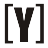# Watts (W) to Amps (A) Calculator

This calculator is very useful for converting watts to amps with easily and quickly.

How to use this calculator first choose Select current type (DC = Direct Current, AC = Alternating Current Single Phase / Three Phase), then fill the Power in Watts, voltage and other values, then press the Calculate button to get the current result in Amps.

 Select current type: DC AC - Single phase AC - Three phase Enter power in watts: W Enter voltage in volts: V Current result in amps: A

## How to Calculate Watts to Amps?

DC = Direct Current
DC (W) to (A) calculation

 Formula I(A) = P(W) V(V)

Current I in amps (A) equals to P in watts (W), divided by voltage V in volts.

AC = Alternating Current
AC single phase (W) to (A) calculation

 Formula I(A) = P(W) (PF x V(V))

AC three phase (W) to (A) calculation
Line to line voltage

 Formula I(A) = P(W) (√3 × PF × VL-L(V) )

Line to neutral voltage

 Formula I(A) = P(W) (3 × PF × VL-N(V) )

power factor of resistive impedance load is equal to 1YoosFuhl.com Would you like to receive notifications on latest updates? No Yes